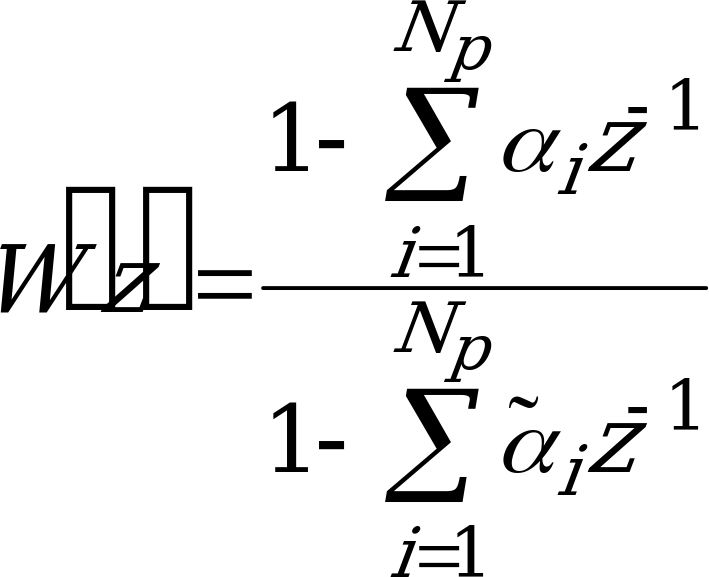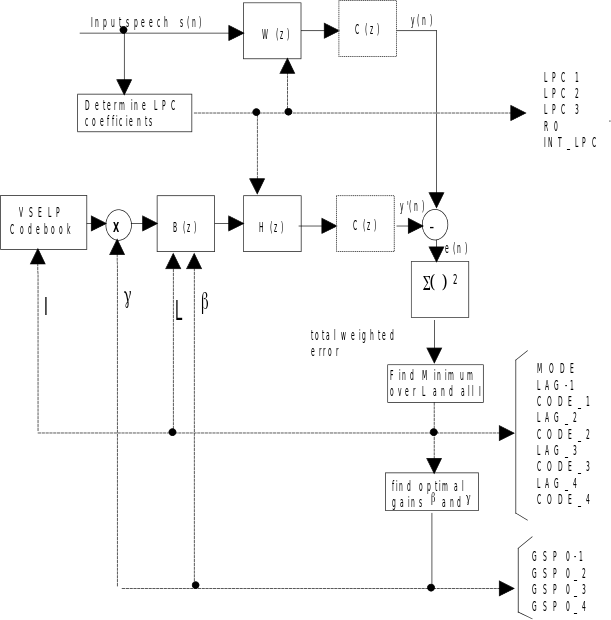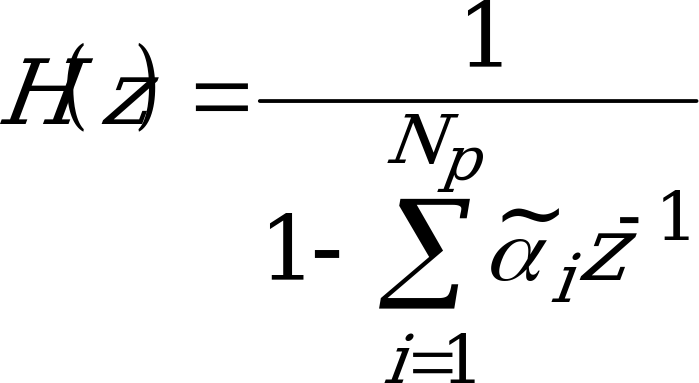## 4.1 GSM half rate speech encoder

06.203GPPHalf rate speech transcodingTS

The GSM half rate speech encoder uses an analysis by synthesis approach to determine the code to use to represent the excitation for each subframe. The codebook search procedure consists of trying each codevector as a possible excitation for the Code Excited Linear Predictive (CELP) synthesizer. The synthesized speech s'(n) is compared against the input speech and a difference signal is generated. This difference signal is then filtered by a spectral weighting filter, W(z), (and possibly a second weighting filter, C(z)) to generate a weighted error signal, e(n). The power in e(n) is computed. The codevector which generates the minimum weighted error power is chosen as the codevector for that subframe. The spectral weighting filter serves to weight the error spectrum based on perceptual considerations. This weighting filter is a function of the speech spectrum and can be expressed in terms of the a parameters of the short term (spectral) filter.(1)

The computation of thei coefficients is described in subclause 4.1.7.

The second weighting filter C(z), if used, is a harmonic weighting filter and is used to control the amount of error in the harmonics of the speech signal. If the weighting filter(s) are moved to both input paths to the subtracter, an equivalent configuration is obtained as shown in figure 2.Figure 2: Block diagram of the GSM half rate speech encoder (MODE = 1,2 and 3)

Here H(z) is the combination of A(z), the short term (spectral) filter, and W(z), the spectral weighting filter. These filters are combined since the denominator of A(z) is cancelled by the numerator of W(z).(2)

There are two approaches that can be used for calculating the gain, . The gain can be determined prior to codebook search based on residual energy. This gain would then be fixed for the codebook search. Another approach is to optimize the gain for each codevector during the codebook search. The codevector which yields the minimum weighted error would be chosen and its corresponding optimal gain would be used for . The latter approach generally yields better results since the gain is optimized for each codevector. This approach also implies that the gain term needs to be updated at the subframe rate. The optimal code and gain for this technique can be computed as follows:

The input speech is first filtered by a high pass filter as described in subclause 4.1.1. The short term filter parameters are computed from the filtered input speech once per frame. A fast fixed point covariance lattice technique is used. Subclauses 4.1.3 and 4.1.4 describes in detail how the short term parameters are determined and quantized. An overall frame energy is also computed and coded once per frame. Once per frame, one of the four voicing modes is selected. If MODE¹0, the long term predictor is used and the long term predictor lag, L, is updated at the subframe rate. L and a VSELP codeword are selected sequentially. Each is chosen to minimize the weighted mean square error. The long-term filter coefficient, , and the codebook gain, , are optimized jointly. Subclause 4.1.8 describes the technique for selecting from among the voicing modes and, if one of voiced modes is chosen, determining the long-term filter lag. Subclause 4.1.10 describes an efficient technique for jointly optimizing ,  and the codeword selection. Subclause 4.1.10 also includes the description of the fast VSELP codebook search technique. The  and  parameters are transformed to equivalent parameters using the frame energy term, and are vector quantized every subframe. The coding of the frame energy and the and  parameters is described in subclause 4.1.11.